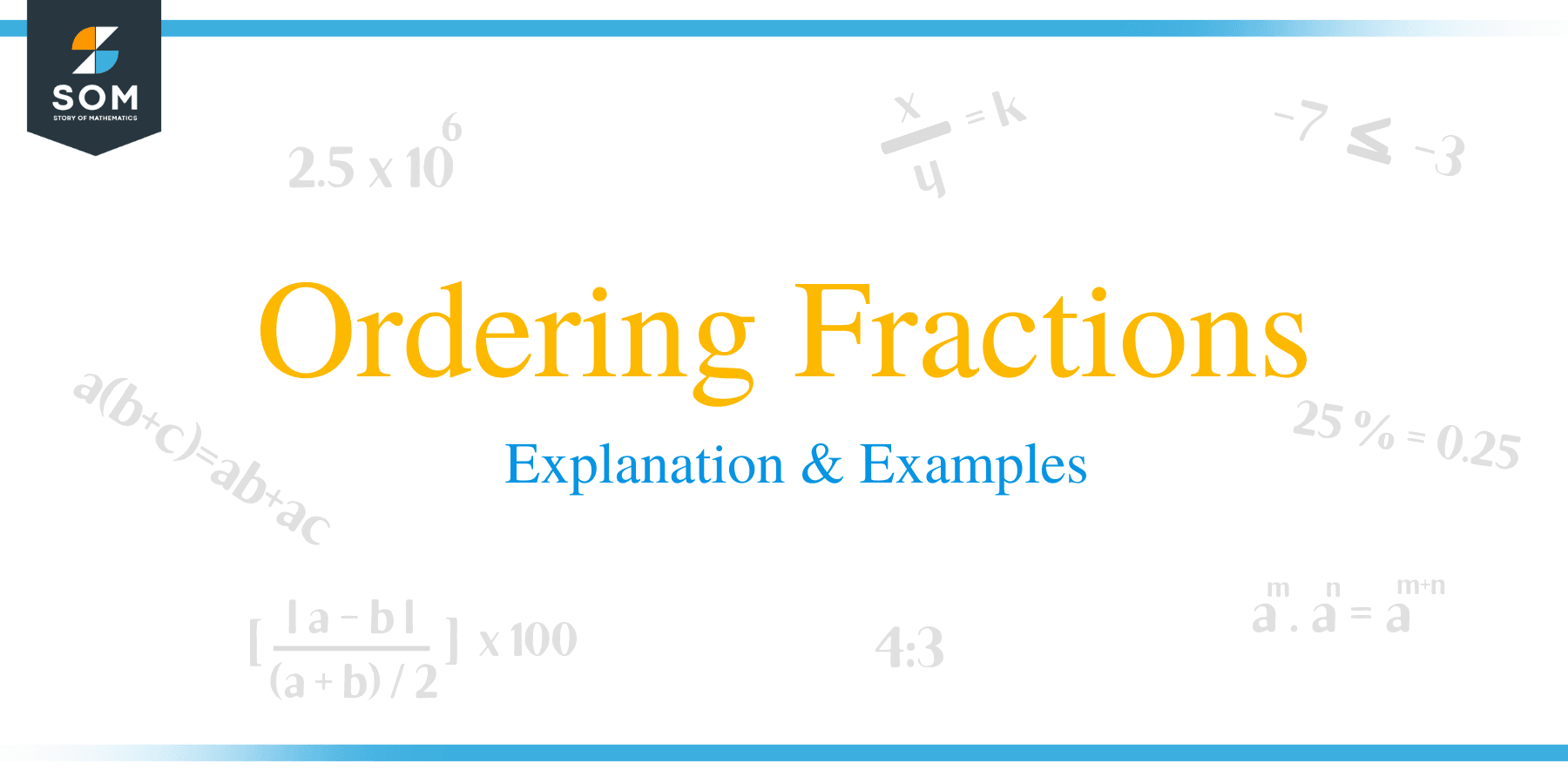# Ordering Fractions – Explanation & Examples

##How to Order Fractions?

Ordering fraction means arranging fractions from the smallest to the largest (ascending order) or largest to smallest (descending order).

There are two common methods of ordering fractions.

These are:

• Using a common denominator.
• Changing fraction to decimals and then ordering.

### Ordering Fractions using Common Denominator

Fractions can be compared and ordered by determining their equivalent fractions with the common denominator. Common denominators are created by using common multiples of the two numbers. For example, 24 is the least common multiple of 8 and 12.

8 x 3 = 24

12 x 2 = 24

Yet 8 and 12 have several other common multiples; however, 24 is the lowest.

### Changing Fractions to Decimals and then Ordering

Conversion of fractions to decimals is another method of ordering fractions.

Example 1

Arrange the following fractions in ascending order.

3/4, 1/2, 4/5, 3/8

Solution

First convert all the fraction to decimals as shown below:

3/4 = 0.75

1/2 = 0.5

4/5 = 0.8

3/8 = 0.375

Since all the fractions have zero in their unit’s digit, compare them by checking the tenths digit.

Now arrange the decimals in descending order.

0.8, 0.75, 0.5, 0.375,

There the final answer is 4/5, 3/4, 1/2 and 3/8

There are also other methods of ordering fractions, such as calculating their percentages.For example, we can solve the problem by expressing it as a percentage.

Order 1/10, 1/5, 1/4, 1/2, 1/3

 Fraction Decimal Percentage 1/10 0.1 10% 1/5 0.2 20% 1/4 0.25 25% 1/2 0.5 50% 1/3 0.3¯ 33.3¯%

Ordering Fractions from Least to Greatest (h2)

Let’s understand this with the help of examples.

Example 2

Arrange the following fractions in ascending order:

1/2, 2/3, 7/12, 5/6, 1/4

Solution

• First, identify all the denominators of the fractions. And in this case, the denominators are 2, 3, 12, 6, and 4.
• Calculate the least common multiple of all the denominators. You look at the L.C.M. of two numbers at a time and check whether the other denominators are factors of the calculated L.C.M.
• The least common multiple of denominators 2, 3, 12, 6 and 4 is 12
• The next step is to rewrite each fraction as an equivalent fraction with the denominator 12.

1/2 x 6/6 = 6/12

2/3 x 4/4 = 8/12

7/12 x 1/1 = 7/12

5/6 x 2/2 = 10/12

1/4 x 3/3 = 3/12

Now that all the fraction shares a common denominator, it is easier to place the fractions in ascending order by comparing their numerators.

By comparing the numerators, the final answer becomes 1/4, 1/2, 7/12, 2/3, 5/6.

More Examples

1.Arrange the following in ascending order:

1/2, 1/4, 3/4

Solution

Find the LCM of 2, 4 which is 4

Multiply 1/2 = 1/2 × 2/2 = 2/4

Since the numerator 4 is remaining in all fractions, order the fraction as follows:

1/4 < 1/2 < 3/4

2. Arrange the fractions below in ascending order:

3/5, 3/7, 9/25

Solution

Determine the LCM of 5, 7 and 25 which is 175

Multiply each fraction by the LCM as:

3/5 = 3/5 × 35/35 = 105/175

3/7 = 3/7 × 25/25 = 75/175

9/25 = 9/25 × 7/7 = 63/175

Now arrange the fractions in ascending order as:

9/25, 3/7, 3/5

3. Order the fraction from the least to the largest.

2/5, 4/7, 5/6

Solution

Find the LCM of 5, 7 and 6 = 210

2/5 = 2/5 × /42/42 = 84/210

4/7 = 4/7 × 30/30 =120/210

5/6 = 5/6 × 35/35 = 175/210

Now fractions in ascending order = 2/5 < 4/7 < 5/6

4. Order the following fractions in ascending order

1/3, 6/9, 9/18

Solution

Determine the LCM of the denominators as 18.

1/3 = 1/3 × 6/6 = 6/18

6/9 = 6/9 × 2/2 = 12/18

Now,

6/18 < 9/18< 12/18 and thus the fraction in ascending order;

1/3 < 9/18 < 6/9

5. Order the fractions below from the lowest to the largest.

3/9, 9/25, 5/20

Solution

Start by calculating the LCM of denominators 4, 20 and 25 = 100

3/4 = 3/4 × 25/25 = 75/100

9/25 = 9/25 × 4/4 = 36/100

5/20 = 5/20 × 5/5 = 25/100

Thus;

25/100 < 36/100 < 75/100

Therefore, the fraction from lowest to largest is

5/20 < 9/25 < 3/4

6. Order these fractions in ascending order:

2/15, 3/18, 9/10

Solution

Calculate the LCM of the denominators 15, 18 and 10 as 90

2/15 = 2/15 × 6/6 = 12/90

3/18 = 3/15 × 5/5 = 15/90

9/10 = 9/10 × 9/9 = 81/90

And thus, the fractions in ascending order are: 2/15 < 3/18 < 9/10

7. List the following fractions in ascending order

16/15, 15/14,14/12

Solution

Calculate the LCM of 15, 14 and 12 as 420

16/15 = 16/15 × 28/28 = 448/420

15/14 = 15/14 × 30/30 = 450/420

14/12 = 14/12 × 35/35 = 490/420

Thus,

448/420 < 450/420 <4 90/4200420 And hence the fractions in ascending order:

16/15 < 15/14 < 14/12

8. Order these fractions in ascending order:

2/3, 3/4, 4/5

Solution

Start by calculating the LCM of the denominators 3, 4 and 5 as 60

2/3 = 2/3× 20/20 = 40/60

3/4 = 3/4 × 15/15 = 45/60

4/5 = 4/5 × 12/12 = 48/60

Now arrange the fractions as:

40/60 < 45/60 < 48/60 And thus, the fractions from the smallest to largest are:

2/3 < 3/4 < 4/5

### Practice Questions

1. Arrange the following fractions in ascending order.

$\left\{\dfrac{4}{5}, \dfrac{6}{7}, \dfrac{11}{14}, \dfrac{3}{20}\right\}$

2. Arrange the following fractions in descending order.

$\left\{\dfrac{4}{5}, \dfrac{6}{7}, \dfrac{11}{14}, \dfrac{3}{20}\right\}$

3. Arrange the following fractions in ascending order.

$\left\{\dfrac{5}{6}, \dfrac{1}{2}, \dfrac{4}{9}, \dfrac{6}{7}\right\}$

4. Arrange the following fractions in descending order.

$\left\{\dfrac{2}{3}, \dfrac{6}{13}, \dfrac{8}{9}, \dfrac{1}{2}\right\}$

5. Arrange the following fractions in ascending order.

$\left\{\dfrac{1}{4}, \dfrac{3}{5}, \dfrac{9}{10}, \dfrac{3}{7}\right\}$

6. Arrange the following fractions in descending order.

$\left\{\dfrac{6}{11}, \dfrac{2}{5}, \dfrac{7}{9}, \dfrac{1}{4}\right\}$